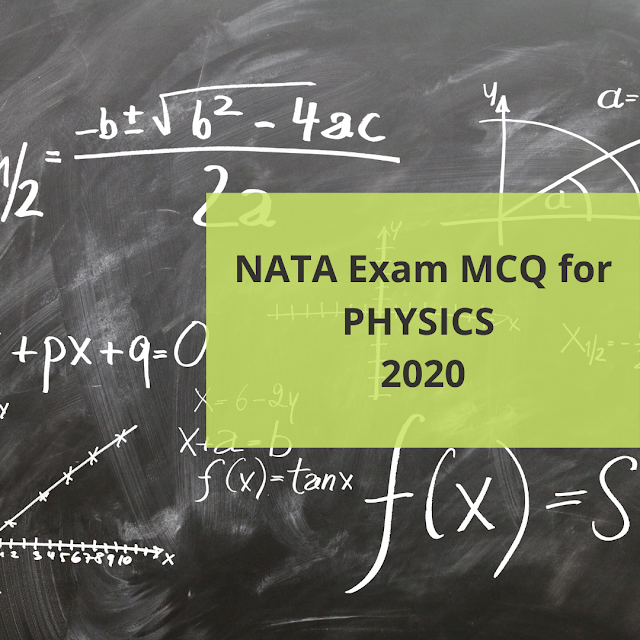## NATA Exam MCQ for PHYSICS (part-2)https://www.fdcareer.in/

1. A conductor with a positive charge.

a) Is always at positive potential

b) Is always at zero potential

c) Is always at negative potential

d) May be at + ve, zero or – ve potential

2. 64 drops each having the capacity C and potential V are combined to form a big drop. if the charge on the small drop is q, then the charge on the big drop will be

a) 2q

b) 4q

c) 16q

d) 64q

3. For measurement of potential difference, a potentiometer is preferred over voltmeter because

a) Potentiometer is more sensitive than
voltmeter

b) The resistance of potentiometer is less
than voltmeter

c) Potentiometer is cheaper than voltmeter

d) Potentiometer does not take current
from the circuit.

4. A Daniel cell is balanced on 125cm length of a potentiometer wire. Now the cell is shortcircuited by a resistance 2 ohm and the balance is obtained at 100cm. The internal resistance of the Daniel cell is

a) 0.5 ohm

b) 1.5 ohm

c) 1.25 ohm

d) 4/5 ohm

5. When there is an electric current through a conducting wire along its length then an electric field must exist

a) Outside the wire but normal to it

b) Outside the wire but parallel to it

c) Inside the wire but parallel to it

d) Inside the wire but normal to it

6. In a cyclotron, a charged particle

a) Undergoes acceleration all the time.

b) Speed up between the does because of
the magnetic field

c) Speeds up in a dee

d) Slow down within a dee and speeds up
between dees

7. A cubical region of space is filled with some uniform electric and magnetic field. An electron enters the cube across one of its faces with velocity V, and a positron enters via opposite face with velocity –V. At this instant

a) The electric forces on both the particles
cause identical acceleration

b) The magnetic forces on both the
particles causes equal acceleration

c) Only electron gains or losses energy

d) The motion of the center of mass is
determined by E alone

8. If a charged particle moves through a magnetic field perpendicular to it

a) Both momentum and energy of particle
charge

b) Momentum as well as energy are
constant

c) Energy is constant but momentum
changes

d) Momentum is constant but energy
changes

9. A current loop placed in a non-uniform magnetic field experience.

a) A force of repulsion

b) A force of attraction

c) A torque but not force

d) A force and a torque

10. If the beams of electrons and protons move parallel to each other in the same direction,
then they

a) Attracts each other

b) Repel each other

c) No relation

d) Neither attract nor repel